## Visualize Linear System at Multiple Simulation Snapshots

This example shows how to visualize linear system characteristics of a nonlinear Simulink® model at multiple simulation snapshots.

1. Open Simulink model.

For example:

`watertank`
2. Open the Simulink Library Browser. In the Simulink Editor, on the Simulation tab, click Library Browser.

3. Add a plot block to the Simulink model.

1. In the Simulink Control Design library, select Linear Analysis Plots.

2. Drag and drop a block, such as the Gain and Phase Margin Plot block, into the Simulink model window.

The model now resembles the following figure.4. Double-click the block to open the Block Parameters dialog box.

To learn more about the block parameters, see the block reference pages.

5. Specify the linearization I/O points.

The linear system is computed for the Water-Tank System.

Tip

If your model already contains I/O points, the block automatically detects these points and displays them. Click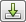at any time to update the Linearization inputs/outputs table with I/Os from the model.

1. To specify an input:

1. Clickadjacent to the Linearization inputs/outputs table.

The Block Parameters dialog expands to display a Click a signal in the model to select it area.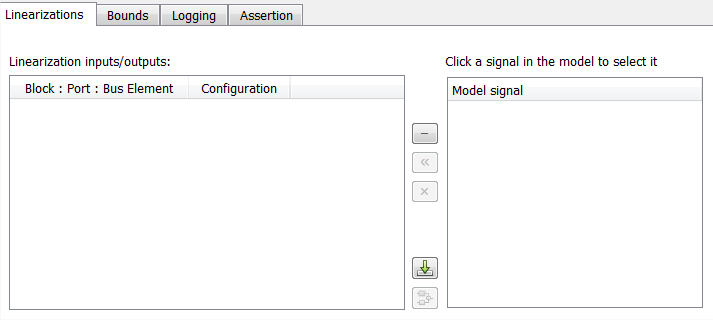2. In the Simulink model, click the output signal of the `PID Controller` block to select it.

The Click a signal in the model to select it area updates to display the selected signal.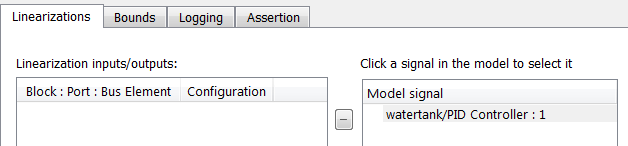Tip

You can select multiple signals at once in the Simulink model. All selected signals appear in the Click a signal in the model to select it area.

3. Click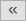to add the signal to the Linearization inputs/outputs table.2. To specify an output:

1. In the Simulink model, click the output signal of the `Water-Tank System` block to select it.

The Click a signal in the model to select it area updates to display the selected signal.2. Clickto add the signal to the Linearization inputs/outputs table.3. In the Configuration drop-down list of the Linearization inputs/outputs table, select `Open-loop Output` for watertank/Water-Tank System : 1.

The Linearization inputs/outputs table now resembles the following figure.3. Clickto collapse the Click a signal in the model to select it area.

Tip

Alternatively, before you add the Linear Analysis Plots block, right-click the signals in the Simulink model and select Linear Analysis Points > Input Perturbation and Linear Analysis Points > Open-loop Output. Linearization I/O annotations appear in the model and the selected signals appear in the Linearization inputs/outputs table.

6. Specify simulation snapshot times.

1. In the Linearizations tab, verify that `Simulation snapshots` is selected in Linearize on.

2. In the Snapshot times field, type `[0 1 5]`.7. Specify a plot type to plot the gain and phase margins. The plot type is `Bode` by default.

1. Select `Nichols` in Plot type

2. Click to open an empty Nichols plot.

8. Save the linear system.

1. Select the Logging tab.

2. Select the Save data to workspace option and specify a variable name in the Variable name field.

The Logging tab now resembles the following figure.9. Plot the gain and phase margins by clicking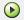in the plot window.

The software linearizes the portion of the model between the linearization input and output at the simulation times of 0, 1 and 5 and plots gain and phase margins.

After the simulation completes, the plot window resembles the following figure.Tip

Clickto view the legend.

The computed linear system is saved as `sys` in the MATLAB® workspace. `sys` is a structure with `time` and `values` fields. To view the structure, type:

`sys`

This command returns the following results:

```sys = time: [3x1 double] values: [4-D ss] blockName: 'watertank/Gain and Phase Margin Plot'```
• The `time` field contains the simulation times at which the model is linearized.

• The `values` field is an array of state-space objects which store the linear systems computed at the specified simulation times.

(If the Simulink model is configured to save simulation output as a single object, the data structure `sys` is a field in the `Simulink.SimulationOutput` object that contains the logged simulation data. For more information about data logging in Simulink, see Export Simulation Data and the `Simulink.SimulationOutput` reference page.)